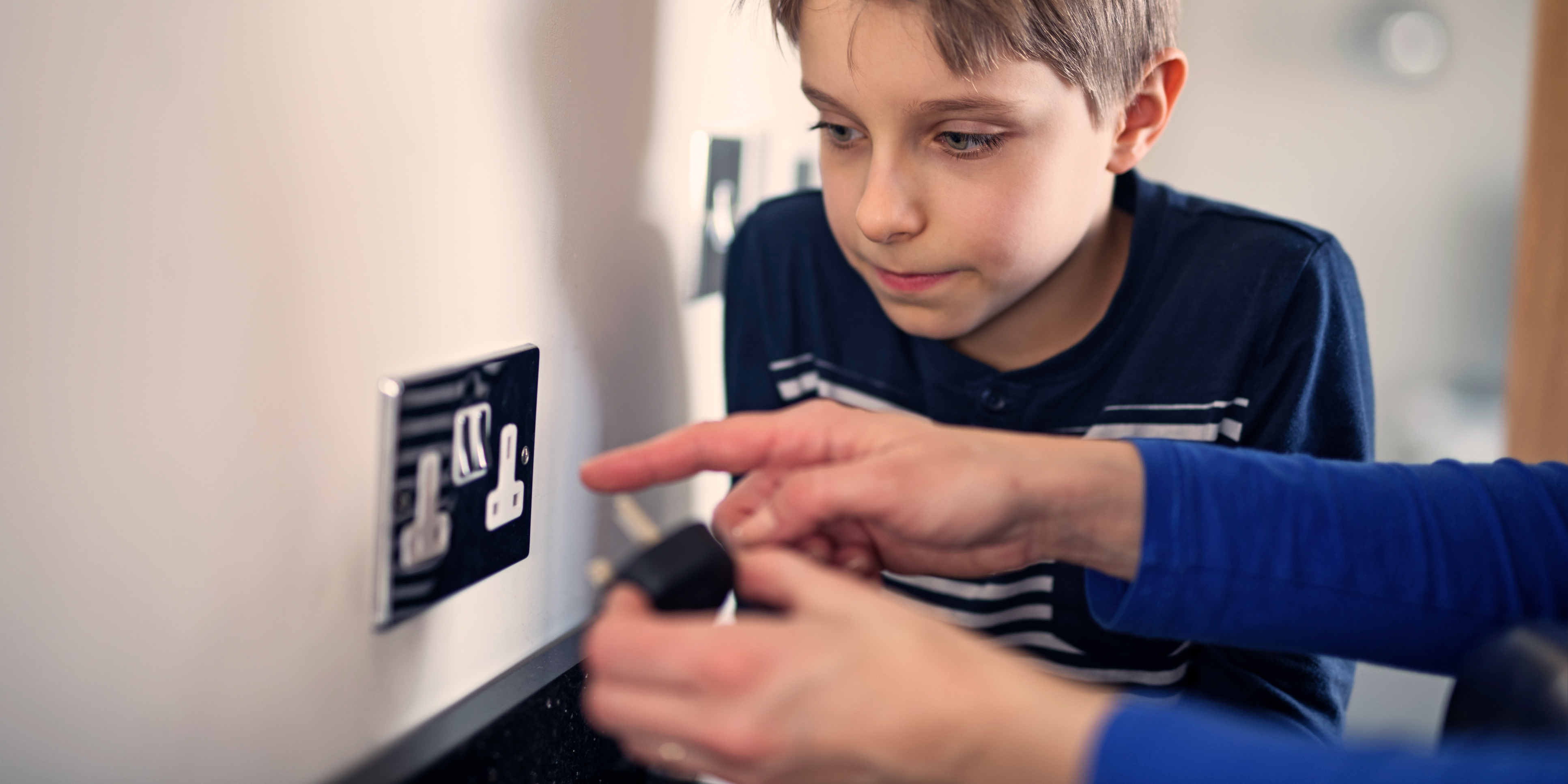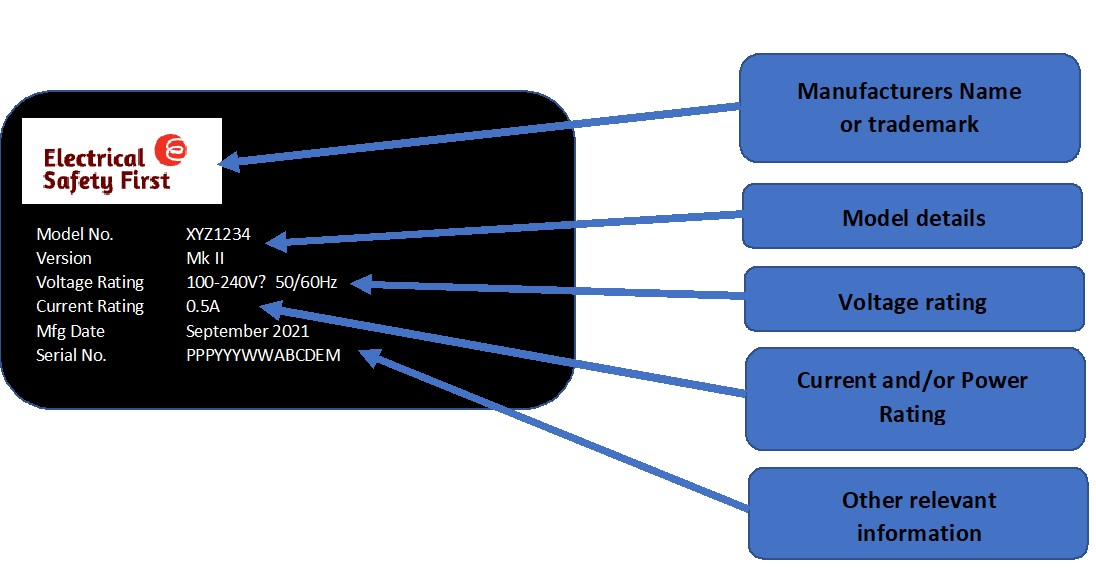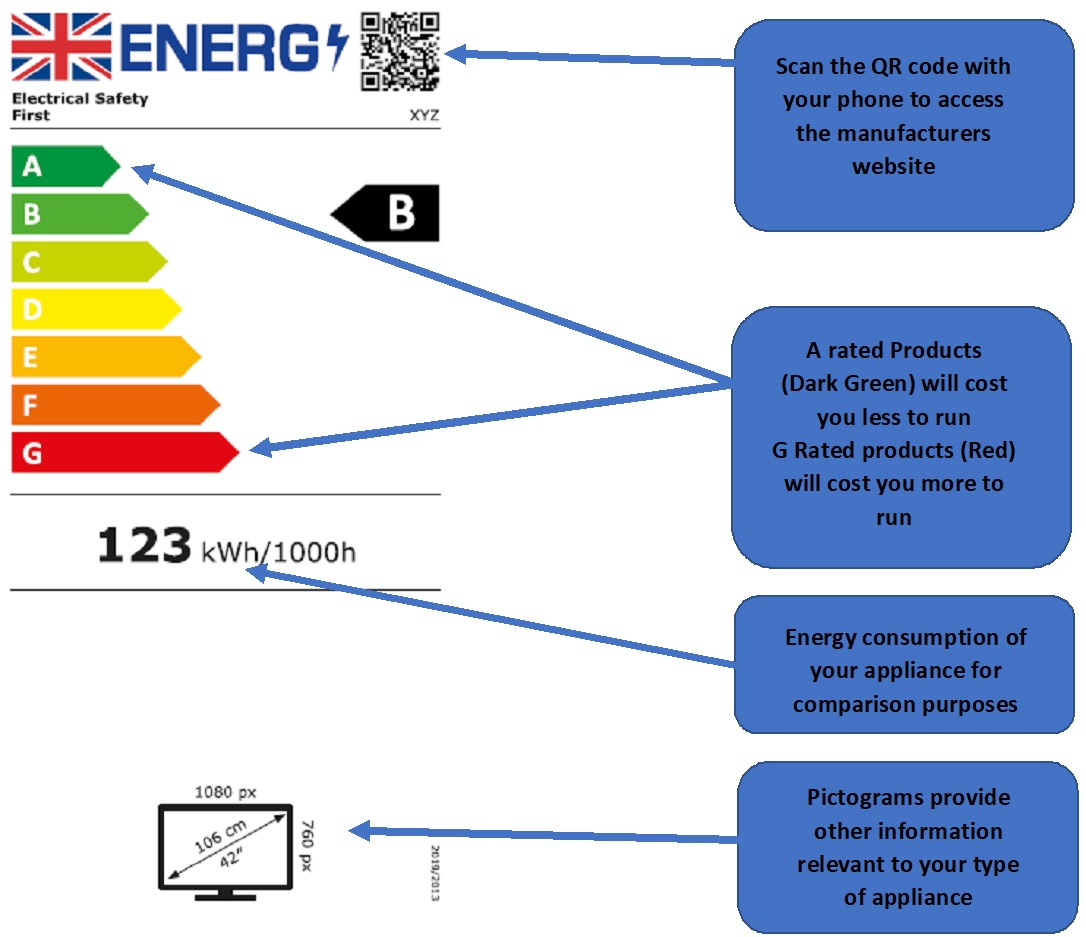# Energy Labels Explained

### What is an energy label and why doesn’t it give me the power of my appliance?

The energy label provides an indication of the energy efficiency (and other key features of products) to help you make an informed choice when making a purchase, and for future reference. However, it may not tell you how much power your appliance uses when you plug it in.

### Can I work out the power of my appliance from the information on the energy label?

It depends. Energy labels will give you the amount of electricity used over a period of time or for a given number of uses (cycles) in kilowatt hours (kWh). To work out the power in watts (W) or kW (kilowatts) of your appliance you need to know how the energy efficiency rating was calculated.  Unfortunately, this calculation differs depending on the type of product.  For example, a TV’s rating is measured in kWh per 1000 hours and a washing machine’s rating is based on kWh per 100 cycles at an eco-wash setting.

If you want to know the power rating of an appliance, it’s easier to look at the rating label on the product or the packaging. See an example of a rating label below.

### How do I work out the power of my appliance from the kWh?

If the power is constant and you know the time frame, it is easy. You just take the kWh and divide it by the number of hours e.g. 10kWh over 5hours = 2kW. If you need the answer in watts (W) instead of kilowatts you just multiply the answer by 1000. e.g. 2 x 1000 = 2000.

Our kilowatt hour (kWh) to watts (W) converter will be coming soon.

### How do I find out the power of my appliance if the power of my appliance is not constant?

You have to look at the rating label. All manufacturers are required to have a rating label on their appliance. This will give you either the power of the appliance or the current of the appliance. You can use our Amps to Watts calculator  or Watts to amp calculator to get the rating information that you need.

### Rating Label Example### Energy Label Example